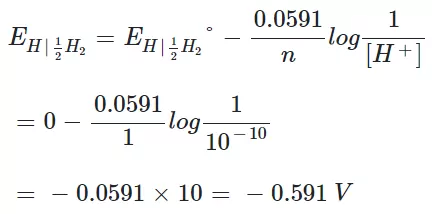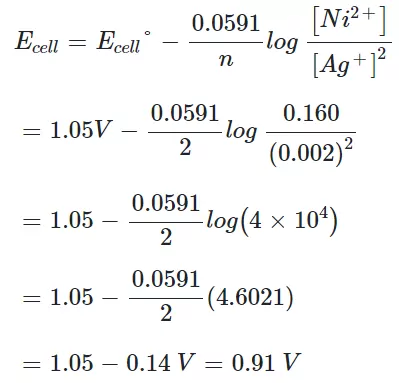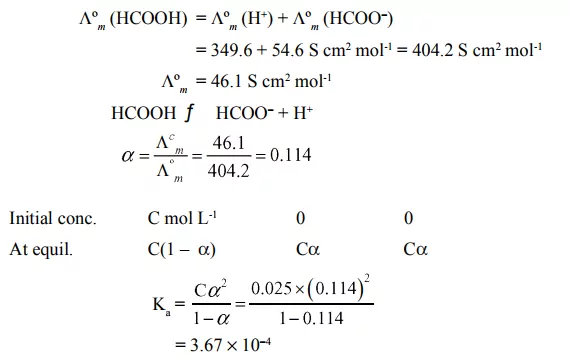# NCERT Intext Questions Class 12 ElectrochemistryNCERT Intext Questions Class 12 Electrochemistry

Que 1. How would you determine the standard electrode potential of the system Mg2+|Mg?
Ans 1. We know that it is not possible to measure the electrode potential of a half cell. We can only measure the difference between electrode potentials of two half cells, which gives the cell potential of the cell. For measuring the standard electrode potential of the given system, we need to use Mg as one electrode and a standard hydrogen electrode as the second electrode. Since the electrode potential of the standard hydrogen electrode is zero, the value of cell potential will give the value of standard electrode potential of the system Mg2+|Mg.
Mg/Mg2+ (aq 1M)//H+(aq 1M)/H2 (1bar), Pt (s)
cell = E°right – E°left
E°left = 0 (The standard hydrogen electrode is always zero)
cell = E°Mg2+|Mg

Que 2. Can you store copper sulphate solutions in a zinc pot?
Ans 2. It is known that zinc is more reactive than copper. Thus if we will store copper sulphate solution in a zinc pot then zinc will displace copper from its solution.
Or
The standard electrode potential of Zinc is – 0.76 whereas that of Copper is 0.34. So Zinc will reduce copper into the lower state.
Zn + CuSO4 → ZnSO4 + Cu

Que 3. Consult the table of standard electrode potentials and suggest three substances that can oxidize ferrous ions under suitable conditions.
Ans 3. Ferrous ion oxidizes to give ferric ions as follows:
Fe2+ → Fe3+ + e
Here, E0 = − 0.77V
It is clear that a substance with a reduction potential greater than 0.77 V can oxidize ferrous ions into ferric ions. Such elements are Br2, Cl2, and F2.

NCERT Intext Questions Class 12 Electrochemistry

Que 4. Calculate the potential of the hydrogen electrode in contact with a solution whose pH is 10.
Ans 4. For hydrogen electrode H+ + e → 12H2
Using Nernst EquationQue 5. Calculate the emf of the cell in which the following reaction takes place:
Ni(s) + 2Ag+ (0.002 M) → Ni2+ (0.160 M) + 2Ag(s) Given that E0(cell) = 1.05 V.
Ans 5. Using Nernst equationQue 6.  The cell in which the following reaction occurs:
2Fe3+ (aq) + 2I– (aq) → 2Fe2+ (aq) + I2 (s) has E°cell = 0.236 V at 298 K. Calculate the standard Gibbs energy and the equilibrium constant of the cell reaction.
Ans 6. For this cell, n = 2
ΔG° = − nFEcell°
= − 2 × 96500 × 0.236 = − 45.55 = − 2 × 96500 × 0.236 = − 45.55 kJ mol-1
Moreover, ΔG° = − 2.303RT log KC
logKC = −ΔG°/2.303RT
= − 45.55/2.303 × 8.314 × 10−3 × 298 = 7.983
Or, KC = antilog(7.983) = 9.616 ×107

NCERT Intext Questions Class 12 Electrochemistry

Que 7. Why does the conductivity of a solution decrease with dilution?
Ans 7. The conductivity of a solution depends upon the number of ions and the distance between them. The number of ions per unit volume of the solution decreases on dilution. Due to this, the conductivity of a solution decreases with dilution.

Que 8. Suggest a way to determine the Λom value of water.
Ans 8. The value of Λ°m can determined by using Kohlrausch’s law as folllows:
Λ°m  = Λ°m (HCl) + Λ°m (NaOH) − Λ°m (NaCl)
Now, Λ°m values of HCl, NaOH and NaCl are substituted in above equation to find the value of Λ°m of water.

Que 9. The molar conductivity of 0.025 mol L-1 methanoic acid is 46.1 S cm2 mol-1. Calculate its degree of dissociation and dissociation constant Given λ°(H+) = 349.6 S cm2 mol-1 and λ°(HCOO) = 54.6 S cm2 mol-1.
Ans 9.Que 10. If a current of 0.5 ampere flows through a metallic wire for 2 hours, then how many electrons would flow through the wire?
Ans 10. Given Current = 0.6 A
Time = 2 hours
We know charge can be calculated using relation:
Q = It
= 0.5 × (2 × 60 × 60) = 3600 C = 0.5 × (2 × 60 × 60) = 3600 C
We know 1F = 96500 C/mol
Moreover, 1 mol of electrons = 6.02 × 1023 electrons
Hence, the number of electrons
= (6.23 × 1023 / 96500) × 3600 = 2.246 × 1022 electrons.

NCERT Intext Questions Class 12 Electrochemistry

Que 11. Suggest a list of metals that can be extracted electrolytically.
Ans 11. Metals like Na, Mg, Al, etc. are produced on a large scale by electrochemical reduction of their respective cations or by the process of electrolysis.
Or
The highly reactive metals that have large -ve E° values, which can themselves act as powerful reducing agents can be extracted electrolytically. The process is known as electrolytic reduction.

Que 12. Consider the reaction: Cr2O72- + 14H+ + 6e→ 2Cr3+ + 7H2O.
What is the quantity of electricity in coulombs needed to reduce 1 mol of Cr2O72- ?
Ans 12. From the reaction, it is clear that 1 mole of Cr2O72- needs 6F of electrical charge
6F = 6×96500 = 579000 C
Therefore 579000 C of electricity is required for the reduction of Cr2O72- to Cr3+.

Que 13. Write the chemistry of recharging the lead storage battery, highlighting all the materials that are involved during recharging.
Ans 13. On charging the battery, the reverse reaction takes place, i.e., PbSO4 deposited on electrodes is converted back to Pb and PbO2 and H2SO4 is regenerated.
At anode: PbSO4 (s) + H+ (aq) + 2e→ Pb (s) + HSO4 (aq)
At cathode: PbSO4 (s) + 2H2O (l) → PbO2 (s) + HSO4 (aq) + 3H+ (aq) + 2e
Overall Reaction:
2PbSO4 (s) + 2H2O (l) → Pb (s) + PbO2 (s) + 2H+ (aq) + 2 HSO4 (aq)

Que 14. Suggest two materials other than hydrogen that can be used as fuels in fuel cells.
Ans 14. Methane (CH4) and methanol (CH3OH) can also be used as fuels in place of hydrogen in the fuel cells.

Que 15. Explain how rusting of iron is envisaged as the setting up of an electrochemical cell.
Ans 15. The water layer present on the surface of iron (especially in the rainy season) dissolves acidic oxides of the air like CO2, SO2, etc. to form acids that dissociate to give H+ ions.
H2O + CO2 → H2CO3
H2CO3 → 2H+ + CO23
In the presence of H+ ions, iron starts losing electrons at some spot to form ferrous ions, i.e., its oxidation takes place. Hence, this spot acts as anode:
Anode: Fe (s) Fe2+ (aq) + 2e
The electrons thus released move through the metal to reach another spot where H+ ions and the dissolved oxygen take up these electrons and a reduction reaction takes place. hence, this spot acts as the cathode:
Cathode: O2 (g) + 4H+ (aq) + 4e 2H2O (l)
The overall reaction is:
2Fe (s) + O2 (g) + 4H+ (aq) → 2Fe2+ (aq) + 2H2O (l)
Thus, an electrochemical cell is set up on the surface.
Ferrous ions are further oxidized by the atmospheric oxygen to ferric ions which combine with water molecules to form a hydrated ferric oxide, Fe2O3.xH2O, which is rust.

NCERT Intext Questions Class 12 Electrochemistry